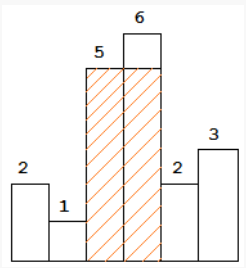New update is available. Click here to update.

# Largest rectangle in a histogram

Last Updated: 29 Dec, 2020
Difficulty: Hard

## PROBLEM STATEMENT

#### You are supposed to return the area of the largest rectangle possible in the given histogram.

##### For example :
``````In the below histogram where array/list elements are {2, 1, 5, 6, 2, 3}.
````````````The area of largest rectangle possible in the given histogram is 10.
``````
##### Input format :
``````The first line contains a single integer ‘T’ denoting the number of test cases.

The first line of each test case contains a single integer ‘N’ denoting the number of elements in the array/list.

The second line contains ‘N’ single space-separated integers denoting the elements of the array/list.
``````
##### Output format :
``````For each test case, print an integer denoting the area of the largest rectangle possible in the given histogram.
``````
##### Note :
``````You do not need to print anything; it has already been taken care of. Just implement the given function.
``````
##### Constraints :
``````1 <= T <= 10
1 <= N <= 10^6
0 <= HEIGHTS[i] <= 10^9

Where ‘T’ is the number of test cases.
'N' is the length of the given array/list.
And, HEIGHTS[i] denotes the height of the 'ith' histogram bar.

Time Limit: 1 sec.
``````## Approach 1

Our intuition is to consider each and every rectangle once so that we can calculate which rectangle has the maximum area.

A simple solution to this problem is to one by one consider all bars as starting points and calculate the area of all rectangles starting with every bar and iterating towards the end of the array/list. Finally, return the maximum of all possible areas.